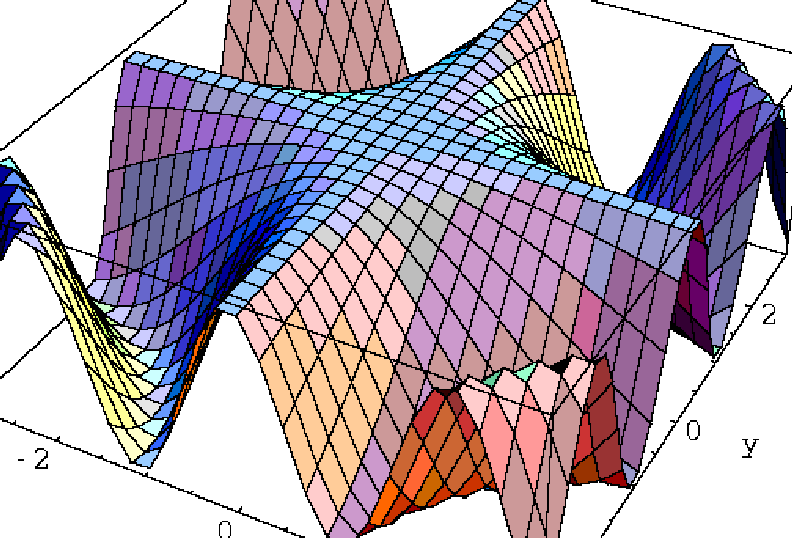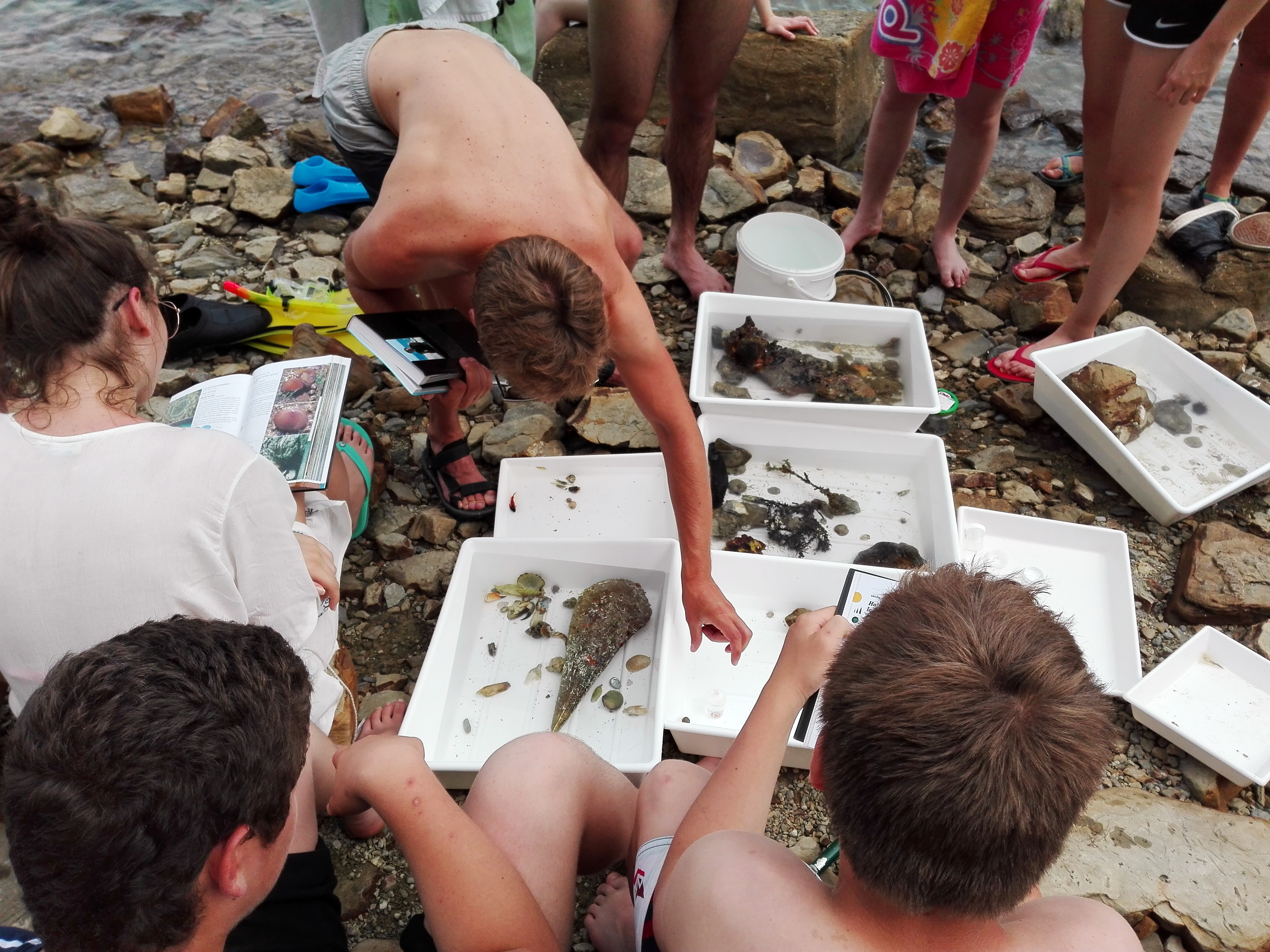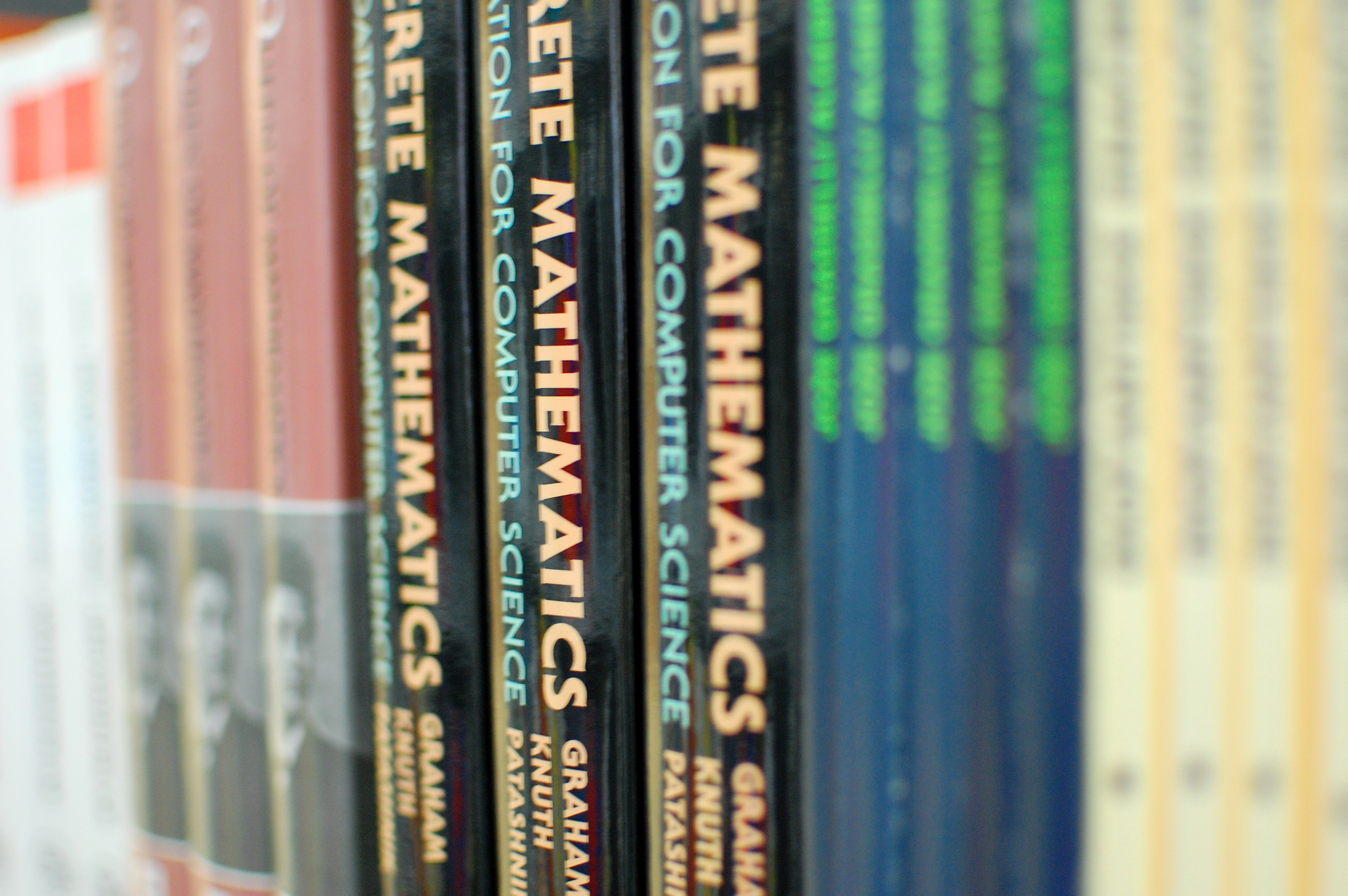Univerza na Primorskem Fakulteta za matematiko, naravoslovje in informacijske tehnologijeSI | EN# Raziskovalni matematični seminar - Arhiv

 2022 2021 2020 2019 2018 2017 2016 2015 2014 2013 2012 2011 2010
 1 2 3 4 5 6 7 8 9 10 11 12
Datum in ura / Date and time: 11.1.21
(10:00 -- 11:00)
Predavalnica / Location: ZOOM (See link below)
Predavatelj / Lecturer: Petr Golovach (Bergen University, Norway)
Naslov / Title: Paths and Cycles Above Combinatorial Bounds
Vsebina / Abstract:

An undirected graph G d-degenerate if every subgraph of G has a vertex of degree at most d, and the degeneracy is the minimum d such that G is d-degenerate. By the classical theorem of Erdös and Gallai from 1959, every graph of degeneracy d>1 contains a cycle of length at least d+1. The proof of Erdös and Gallai is constructive and can be turned into a polynomial time algorithm constructing a cycle of length at least d+1. But can we decide in polynomial time whether a graph contains a cycle of length at least d+2? An easy reduction from Hamiltonian Cycle provides a negative answer to this question: Deciding whether a graph has a cycle of length at least d+2 is NP-complete.  Surprisingly, the complexity of the problem changes drastically when the input graph is 2-connected.  In this case we prove that    deciding whether G contains a cycle of length at least d+k can be done in time 2^{O(k)}|V(G)|^{O(1)}.  Further, we briefly consider similar problem arising from the classical result of Dirac from 1952 that every 2-connected n-vertex graph has a cycle with at least min{2\delta(G),n} vertices.

The talk is based on the joint work with Fedor V. Fomin, Daniel Lokshtanov, Fahad Panolan, Saket Saurabh, Meirav Zehavi, Danil Sagunov, and Kirill Simonov.

We are looking forward to meeting at the video-conference. Join Zoom Meeting HERE!

Everyone is welcome and encouraged to attend.

Datum in ura / Date and time: 4.1.21
(10:00 -- 11:00)
Predavalnica / Location: ZOOM (See link below)
Predavatelj / Lecturer: Ted Dobson (UP FAMNIT, Slovenia)
Naslov / Title: Recognizing vertex-transitive digraphs which are wreath products, double coset digraphs, and generalized wreath products
Vsebina / Abstract:

It is known that a Cayley digraph $\Cay(A,S)$ of an abelian group $A$ is isomorphic to a nontrivial wreath product if and only if there is a proper nontrivial subgroup $B\le A$ such that $S\setminus B$ is a union of cosets of $B$ in $A$. We generalize this result to Cayley digraphs $\Cay(G,S)$ to nonabelian groups $G$ by showing that such a digraph is isomorphic to a nontrivial wreath product if and only if there is a proper nontrivial subgroup $H\le G$ such that $S\setminus H$ is a union of double cosets of $H$ in $G$. This result is proven in the more general situation of a double coset digraph (also known as a Sabidussi coset digraph.) We then give applications of this result which include showing the problem of determining automorphism groups of vertex-transitive digraphs is equivalent to the problem of determining automorphism groups of Cayley digraphs, and extending the definition of generalized wreath product digraphs to double coset digraphs of all groups $G$.

Join Zoom Meeting HERE!

Everyone is welcome and encouraged to attend.